# AWK is awesome

AWK is awesome because it is the most rewarding programing language you can learn for bioinformatics and Data Science. There is little to learn and it is useful every day.

AWK has been the most beneficial programming language I have ever learned. It took me only a day to learn most of it and it saved me several weeks if not months already. I use AWK almost every day.

It is better to see AWK in action once than to hear about it a thousand times. So, let’s start with the examples.

## Table summary

I usually use AWK to calculate some simple summary statistics for a table. For example, let’s assume you have a file `table.txt` with some numeric values:

``````CHR STAR    END LOD SCORE
chr1	211829	211850	lod=31	333
chr1	211867	211871	lod=13	247
chr1	211877	211903	lod=66	408
chr1	211913	211927	lod=61	400
chr1	211971	211994	lod=60	399
chr1	211996	212024	lod=72	417
chr6	310311	310324	lod=16	268
chr6	312061	312066	lod=13	247
chr6	312100	312206	lod=376	580
chr6	312653	312728	lod=19	285
chr6	312908	313028	lod=348	573
chr6	313549	313788	lod=900	667
chr6	313589	313784	lod=747	648
``````

### Mean

You can quickly get the mean `SCORE` value:

``````awk '{s+=\$5} END {print s/(NR-1)}' table.txt
``````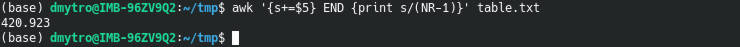where `s+=\$5` sums up all values of the 5th column; `NR` is a built-in variable that equals to the row number. I use `NR-1` because I skip the header. The command after the `END` is executed when the end of the file is reached.

To see what AWK does line by line, run this command:

``````awk 'BEGIN{print "SCORE", "SUM", "LINE_NUMBER"} {s+=\$5; print \$5, s, NR} END {print "mean:", s/(NR-1)}' table.txt
``````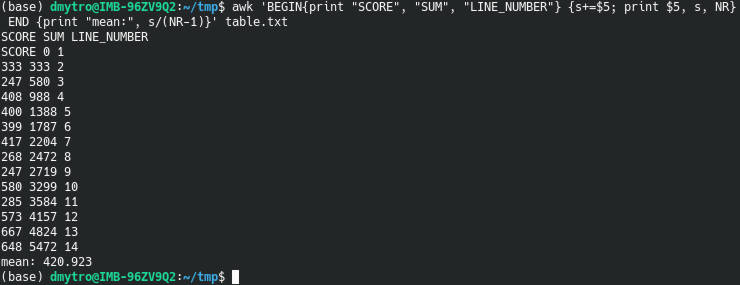But how to calculate the mean of the `LOD` columns that has `lod=` in front of each number?

You can use AWK to clean tha data and do the calculation:

``````awk 'gsub( "lod=", "" , \$4){s+=\$4}END{print s/(NR-1)}' table.txt
``````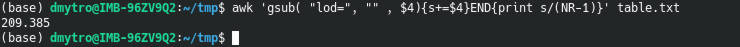`gsub( "lod=", "" , \$4)` replaces `lod=` with an empty string before any calculation is done.

You can also limit the calculation to one chromosome:

``````awk '\$1=="chr1" {n++; s+=\$5} END {print s/n}' table.txt
``````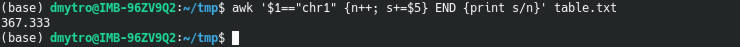We use the condition if (`\$1=="chr1"`) do (`{n++; s+=\$5}`). Also, `NR` is replaced with `n++` to count only the lines that meet the condition `\$1=="chr1"`

### Min and max

Using the same principles, you can get the minimum and maximum values of the `SCORE` column:

``````awk 'NR==2 || \$5 < min {min=\$5} END{ print min}' table.txt
awk 'NR==2 || \$5 > max {max=\$5} END{ print max}' table.txt
``````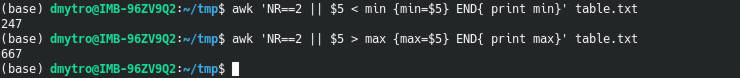`||` means OR statement in AWK.

You can combine these two commands in one:

``````awk 'NR==2 {min=\$5; max=\$5} \$5 > max {max=\$5} \$5 < min {min=\$5} END {print "min: ", min, "\nmax: ", max}' table.txt
``````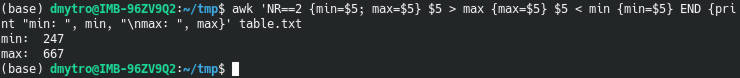`NR==2 {min=\$5; max=\$5}` assigns the initial values of `min` and `max` using the second row. `\$5 > max {max=\$5}` and `\$5 < min {min=\$5}` are conditional statements that are checked one after another.

### Mean, max, and min in one line

You can also combine all three calculations in one line and get all statistics in one run:

``````awk ' NR==2 {min=\$5; max=\$5} \$5 > max {max=\$5} \$5 < min {min=\$5} {s+=\$5} END {print "min: ", min, "\nmax: ", max, "\nmean: ", s/(NR-1)}' table.txt
``````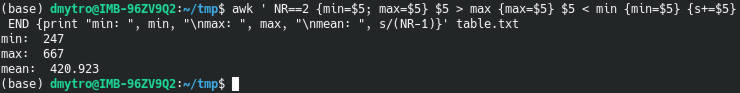## Genotypes summary

There are more complicated cases where you can use AWK.

You may want to do some calculations of the genotype table generated by VariantsToTable from the GATK:

``````#CHROM  POS     REF     12.4.GT 13.16.GT        16.9.GT
scaffold_1      191     A       ./.     ./.     A/A
scaffold_1      563     T       T/T     ./.     T/A
scaffold_1      647     A       C/C     C/C     A/C
scaffold_1      669     T       T/T     T/T     T/T
scaffold_1      679     C       C/A     C/A     C/A
scaffold_1      704     T       T/C     T/C     T/C
scaffold_1      721     T       C/C     C/C     C/C
scaffold_1      722     C       C/T     C/T     C/T
scaffold_1      733     G       G/T     G/T     G/*
``````

For example, I often calculate the number heterozygous, homozygous sites and missing genotypes. To that end, I use this AWK script written in the summarizeTAB.awk file:

``````{if (NF > maxNF ) {
for (i = 4; i <= NF; i++)
countN[i] = 0; countHomo[i] = 0; countHetero[i] = 0; countNA[i] = 0; maxNF = NF;
}
if (NR == 1 ) { for (i = 4; i <= NF; i++) samples[i] = \$i;}
else {
for (i = 3; i <= NF; i++)
{if (\$i == "N" || \$i == "./.") countN[i]++;
else if (\$i == "A/A" || \$i == "T/T" || \$i == "G/G" || \$i == "C/C") countHomo[i]++;
else if (\$i == "G/A" || \$i == "T/C" || \$i == "A/C" || \$i == "G/T" || \$i == "C/G" || \$i == "A/T" || \
\$i == "A/G" || \$i == "C/T" || \$i == "C/A" || \$i == "T/G" || \$i == "G/C" || \$i == "T/A") \
countHetero[i]++;
else countNA[i]++;
}
}
}
END {
print "Sample", "Genotypes", "Heterozygots", "Homozygots", "Missing", "Unknown";
for (i = 4; i <= maxNF; i++)
print samples[i], countHomo[i]+countHetero[i]+0, countHetero[i]+0, countHomo[i]+0,  countN[i]+0, countNA[i]+0;
}
``````

It loops through the columns starting from the 4th one and calculates the number of the number heterozygous, homozygous, missing, and unknown genotypes. These number are stored in corresponding variables.

When the script is too long to fit it in one line as in this case, you can write it into a file and tell AWK to execute it:

``````awk -f summarizeTAB.awk geno.tab
``````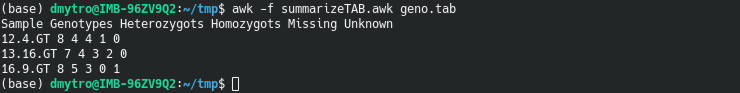## AWK vs Python

The AWK code is usually shorter and works faster than Python. I do not have a dramatic example when my AWK code is substantially shorter than the equivalent Python code. But there are great examples from other AWK users.

## Be careful with AWK

There is one key point you need to keep in mind when you work with AWK. It doesn’t throw an error when it encounters something unusual. Instead, AWK tried to guess how to handle it and proceeds silently. This can put you in danger.

Using the mean `SCORE` column example from above, you can see that AWK treated the characters in the header as `0`.

This would throw you an error in Python. A character string and numeric value cannot be summed. But AWK doesn’t give such an error.

You would have done a mistake in the mean calculation if you calculated the line numbers as `n++`. It would have counted the header too. That’s why I also deduced 1 from the number of rows specified with `NR`.

Similarly, if you have missing data points in a form of `NA`, you need to tell AWK to skip them:

``````awk 'NR>1 && \$5!="NA" {s+=\$5; n++; print \$5, s, n} END {print "mean:", s/n}' table.txt
``````

I also used `NR>1` to skip the header.

So, you need to be aware of this behavior of AWK when you have a mixture of data types.

## Where to learn AWK

If you want to learn AWK, I recommend the course “To awk or not to…”. It was fantastic when I took it in 2017, and it has improved since then.

I also often visit this AWK page, for quick reference on the functions.

If you have never used AWK, give it a try. It may change your life forever.

Written on September 17, 2019